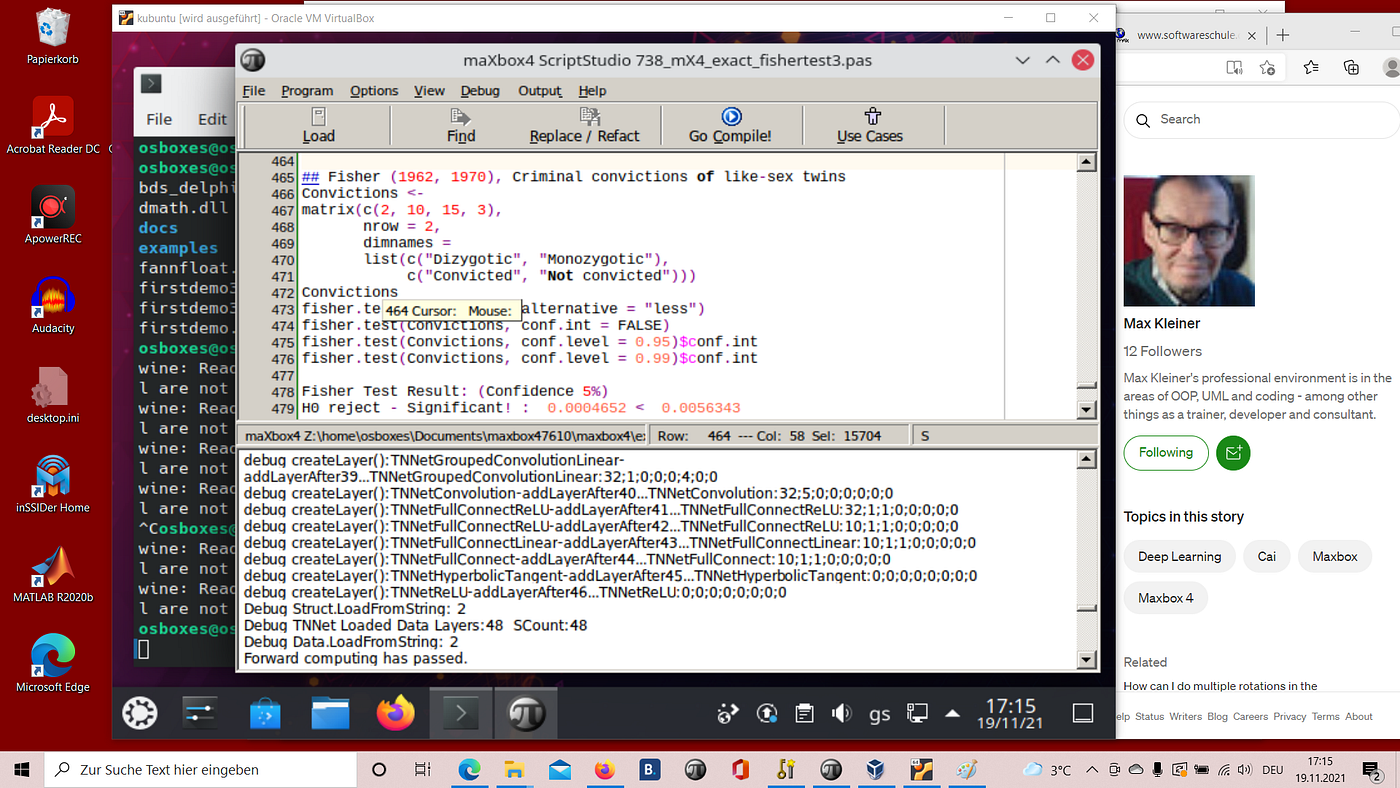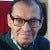# Learning boolean functions XOR, AND and OR with the CAI NeuralNetwork Framework

This example has these main steps:

• Preparing training data
• Creating the neural network
• Fitting
• Printing a test result
• Visualize inference

These are the inputs and expected outputs:

`const inputs : TBackInput = ( // x1, x2 ( 0.1, 0.1), // False, False ( 0.1, 0.9), // False, True ( 0.9, 0.1), // True, False ( 0.9, 0.9) // True, True ); const reluoutputs : TBackOutput = (// XOR, AND, OR ( 0.1, 0.1, 0.1), ( 0.8, 0.1, 0.8), ( 0.8, 0.1, 0.8), ( 0.1, 0.8, 0.8) );`

The first row in `reluoutputs` has expected outputs for XOR, AND and OR boolean functions while the first row of
`inputs` contains inputs for these 3 boolean functions. All 3 boolean functions will be trained together in this example.

This is how the training data is prepared with training pairs (input,output):

`TrainingPairs := TNNetVolumePairList.Create(); ... for Cnt := Low(inputs) to High(inputs) do begin TrainingPairs.Add( TNNetVolumePair.Create( TNNetVolume.Create(vInputs[Cnt]), TNNetVolume.Create(vOutput[Cnt]) ) ); end;`

In this example, values smaller than 0.5 mean false while values bigger than 0.5 mean true. This is called monopolar encoding.

CAI also supports bipolar encoding (-1, +1). Please have a look directly into the source code at these 2 methods:

• `EnableMonopolarHitComparison()`
• `EnableBipolarHitComparison()`

This is how the neural network is created:

`NN := TNNet.Create(); ... NN.AddLayer( TNNetInput.Create(2) ); NN.AddLayer( TNNetFullConnectReLU.Create(3) ); NN.AddLayer( TNNetFullConnectReLU.Create(3) );`

As you can see, there is one input layer followed by 2 fully connected layers with 3 neurons each.

This is how the fitting object is created and run:

`NFit := TNeuralFit.Create(); ... NFit.InitialLearningRate := 0.01; NFit.LearningRateDecay := 0; NFit.L2Decay := 0; NFit.Verbose := false; NFit.HideMessages(); NFit.Fit(NN, TrainingPairs, nil, nil, {batchsize=}4, {epochs=}3000);`

The neural network is then tested for each input with:

`// tests the learning for Cnt := Low(inputs) to High(inputs) do begin NN.Compute(vInputs[Cnt]); NN.GetOutput(pOutPut); WriteLn ( ' Output:', pOutPut.Raw:5:2,' ', pOutPut.Raw:5:2,' ', pOutPut.Raw:5:2, ' - Training/Desired Output:', vOutput[cnt]:5:2,' ', vOutput[cnt]:5:2,' ' , vOutput[cnt]:5:2,' ' ); end;`

# Code in maXbox scripting

`type TBackInput = array[0..3] of array[0..1] of TNeuralFloat; type TBackOutput = array[0..3] of array[0..2] of TNeuralFloat; { const inputs : TBackInput = ( // x1, x2 ( 0.1, 0.1), // False, False ( 0.1, 0.9), // False, True ( 0.9, 0.1), // True, False ( 0.9, 0.9) // True, True ); const reluoutputs : TBackOutput = (// XOR, AND, OR ( 0.1, 0.1, 0.1), ( 0.8, 0.1, 0.8), ( 0.8, 0.1, 0.8), ( 0.1, 0.8, 0.8) ); } procedure DefinelogicalMatrix(var inputs:TBackInput; var routput:TBackOutput); begin Inputs:= 0.1; Inputs:= 0.1; Inputs:= 0.1; Inputs:= 0.9; Inputs:= 0.9; Inputs:= 0.1; Inputs:= 0.9; Inputs:= 0.9; routput:= 0.1; routput:= 0.1; routput:= 0.1; routput:= 0.8; routput:= 0.1; routput:= 0.8; routput:= 0.8; routput:= 0.1; routput:= 0.8; routput:= 0.1; routput:= 0.8; routput:= 0.8; end; procedure RunSimpleAlgo(); var NN: TNNet; EpochCnt: integer; Cnt: integer; pOutPut: TNNetVolume; vInputs: TBackInput; vOutput: TBackOutput; inputs: TBackInput; routput : TBackOutput; Rate, Loss, ErrorSum : TNeuralFloat; begin definelogicalMatrix(inputs, routput); NN := TNNet.Create(); NN.AddLayer( TNNetInput.Create3(2) ); NN.AddLayer( TNNetFullConnectReLU.Create30(3,0) ); NN.AddLayer( TNNetFullConnectReLU.Create30(3,0) ); NN.SetLearningRate(0.01, 0.9); vInputs := inputs; vOutput := routput; //constructor Create(pSizeX, pSizeY, pDepth: integer; c: T = 0); {\$IFNDEF FPC} overload; {\$ENDIF} pOutPut := TNNetVolume.Create0(3,1,1,1); for EpochCnt := 1 to 4000 do begin for Cnt := Low(inputs) to High(inputs) do begin NN.Compute68(vInputs[Cnt],0); NN.GetOutput(pOutPut); NN.Backpropagate70(vOutput[Cnt]); if EpochCnt mod 300 = 0 then WriteLn ( itoa(EpochCnt)+' x '+itoa(Cnt)+ ' Output:'+ format(' %5.2f',[poutPut.Raw])+' '+ format(' %5.2f',[poutPut.Raw])+' '+ format(' %5.2f',[poutPut.Raw])+' '+ (* pOutPut.Raw:5:2,' ', pOutPut.Raw:5:2, *) ' - Training/Desired Output:'+ format('%5.2f',[vOutput[cnt]])+' '+ format('%5.2f',[vOutput[cnt]])+' '+ format('%5.2f',[vOutput[cnt]])+' ' {vOutput[cnt]:5:2,' ', vOutput[cnt]:5:2,' ' , vOutput[cnt]:5:2,' ' } ); end; if EpochCnt mod 300 = 0 then WriteLn(''); end; // TestBatch( NN, pOutPut, 10000, Rate, Loss, ErrorSum); //NN.DebugWeights(); NN.DebugErrors(); pOutPut.Free; NN.Free; Write('Press ENTER to exit.'); //ReadLn; end;`

# Output:

Ref: simple logical learner
300 x 0 Output: 0.30 0.07 0.26 — Training/Desired Output: 0.10 0.10 0.10
300 x 1 Output: 0.40 0.29 0.62 — Training/Desired Output: 0.80 0.10 0.80
300 x 2 Output: 0.72 0.22 0.62 — Training/Desired Output: 0.80 0.10 0.80
300 x 3 Output: 0.37 0.56 1.00 — Training/Desired Output: 0.10 0.80 0.80

# Visualize XOR

Its not always so clear as we do reasoning.

If you assume that:
0.1 = False.
0.8 = True.
(0.1+0.8) = 0.45 = Threshold.
y < 0.45 = False.
y > 0.45 = True.

With above assumptions, you’ll find that your “others very different” results are precise results in boolean terms.

This API implements Stochastic Gradient Descent. As the name implies, it’s not deterministic. Most probably, if you increment the number of epochs, results will look more stable.

Layer 0 Max Error: 0 Min Error: 0 Max ErrorD: 0 Min ErrorD: 0 TNNetInput 2,1,1
debug errors else
Layer 1 Max Error: 0.000858666782733053 Min Error: -0.00092624151147902 Max ErrorD: 0 Min ErrorD: 0 TNNetFullConnectReLU 3,1,1
Parent:0
Layer 2 Max Error: 0.0012739896774292 Min Error: -0.00215935707092285 Max ErrorD: 0 Min ErrorD: 0 TNNetFullConnectReLU 3,1,1
Parent:1
Press ENTER to exit.

We can also visualize the decision boundary :

# Visualise Neurons

It’s usually very hard to understand neuron by neuron how a neural network dedicated to image classification internally works.
One technique used to help with the understanding about what individual neurons represent is called Gradient Ascent.

In this technique, an arbitrary neuron is required to activate and then the same backpropagation method used for learning is
applied to an input image producing an image that this neuron expects to see.

To be able to run this example, you’ll need to load an already trained neural network file (for example https://sourceforge.net/projects/maxbox/files/Examples/CAI/1076_SimpleImageClassifierEKON25_5000.nn/download) and then select the layer you intend to visualize.

Deeper convolutional layers tend to produce more complex patterns. Above image was produced from a very first convolutional layer. The following image was produced from a third convolutional layer. Notice that patterns are more complex.

This is the API method used for an arbitrary neuron backpropagation (Gradient Ascent):

`procedure TNNet.BackpropagateFromLayerAndNeuron(LayerIdx, NeuronIdx: integer; Error: TNeuralFloat);`

Errors on the input image aren’t enabled by default. In this example, errors regarding the input image are enabled with this:

`TNNetInput(FNN.Layers).EnableErrorCollection();`

Then, errors are added to the input with this:

`vInput.MulAdd(-1, FNN.Layers.OutputError); FNN.ClearDeltas(); FNN.ClearInertia();`

You can find more about Gradient Ascent at:

As we know about 50 epochs deliver useful results with the CIFAR10 image set:

Starting Testing.
Epochs: 50 Examples seen:2400000 Test Accuracy: 0.8481 Test Error: 0.4238 Test Loss: 0.4620 Total time: 188.44min
Epoch time: 3.2887 minutes. 50 epochs: 2.7406 hours.
Epochs: 50. Working time: 3.14 hours.
Starting Testing.
Epochs: 50 Examples seen:2400000 Test Accuracy: 0.8481 Test Error: 0.4238 Test Loss: 0.4620 Total time: 189.71min
Finished.

Last point concerning correlations: Correlations are useful because they can indicate a predictive relationship that can be exploited in practice. For example, an electrical utility may produce less power on a mild day based on the correlation between electricity demand and weather. In this example, there is a causal relationship, because extreme weather causes people to use more electricity for heating or cooling. However, in general, the presence of a correlation is not sufficient to infer the presence of a causal relationship (i.e., correlation does not imply causation).

You can find the scripts at_:

https://sourceforge.net/projects/maxbox/files/Examples/CAI/

Formally, random variables are dependent if they do not satisfy a mathematical property of probabilistic independence. In informal parlance, correlation is synonymous with dependence.deep learning on kubuntu with maXbox 4.7.6

Originally published at http://maxbox4.wordpress.com on November 18, 2021.

--

--## Max Kleiner

Max Kleiner's professional environment is in the areas of OOP, UML and coding - among other things as a trainer, developer and consultant.